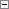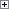Deletes one or more characters in a string.

NewString := DELCHR(String[, Where][, Which])

Parameters

String

Type: Text constant or code

The input string.

Where

Type: Text constant or code

Specifies where to delete characters.

You can include one or more of the following characters in the Where string:

• =   To delete any character in String that matches a character in Which (default)
• <   To delete any leading character in String that matches a character in Which
• >   To delete any trailing character in String that matches a character in Which
Which

Type: Text or code

Specifies the characters that you want to delete.

The default is a space character.Property Value/Return Value

Type: Text constant or code

The resulting string.Remarks

The DELCHR function is case-sensitive.

If you omit the Which parameter, then the function deletes spaces from String based on the contents of the Where parameter as follows:

• If Where contains =, then all the spaces are deleted from String.
• If Where contains <, then all the spaces at the start of String are deleted.
• If Where contains >, then all the spaces at the end of String are deleted.
• If Where contains any other character, then an error is returned.
• If Where is empty, then String is returned unchanged.

If you use the Where and the Which parameters, then the function deletes from String the characters that are contained in the Which parameter based on the contents of the Where parameter as follows:

• If Where contains =, then every occurrence of the characters in Which are deleted from String.
• If Where contains <, then the characters in Which are only deleted if they occur at the start of String.
• If Where contains >, then the characters in Which are deleted only if they occur at the end of String.
• If Where contains any other character, then an error is returned.
• If Where is empty, then String is returned unchanged.
• If Which is empty, then String is returned unchanged.

The Which parameter contains an array of the characters that you want to delete. The order of the characters is of no significance. If String contains a character that is specified in Which, it is deleted from String.Example

This example requires that you create the following variables and text constants in the C/AL Globals window.

Variable name DataType

String

Text

Where

Text

Which

Text

NewString

Text

Text constant name ConstValue

Text000

Windy West Solutions

Text001

>%1< is transformed to >%2<Copy Code
String := Text000;
Where := '<>';
Which := 'Ws';
NewString := DELCHR(String, Where, Which);
MESSAGE(Text001, String,NewString);

The message window displays the following:

>Windy West Solutions< is transformed to >indy West Solution<

The function deletes every W and s that is either the first or last character in String.Example

This example requires that you create the following variables and text constants in the C/AL Globals window.

Variable name DataType

String

Text

Where

Text

Which

Text

NewString

Text

Text constant name ConstValue

Text000

This is an example

Text001

>%1< is transformed to >%2<Copy Code
String := Text000;
Where := '=';
Which := 'sx';
NewString := DELCHR(String, Where, Which);
MESSAGE(Text001, String,NewString);

The message window displays the following:

>This is an example< is transformed to >Thi i an eample<

The function deletes every s and x from String.Example

This example requires that you create the following variables and text constants in the C/AL Globals window.

Variable name DataType

String

Text

Where

Text

Which

Text

NewString

Text

Text constant name ConstValue

Text000

This is an example

Text001

>%1< is transformed to >%2<Copy Code
String := Text000;
Where := '>';
Which := 'Tely';
NewString := DELCHR(String, Where, Which);
MESSAGE(Text001, String,NewString);

The message window displays the following:

>This is an example< is transformed to >This is an examp<

If T, e, l, or y is the last character in String, the function deletes them.Example

This example requires that you create the following variables and text constants in the C/AL Globals window.

Variable name DataType

String

Text

Where

Text

Which

Text

NewString

Text

Text constant name ConstValue

Text000

This is an example

Text001

>%1< is transformed to >%2<Copy Code
String := Text000;
Where := '<';
Which := 'This';
NewString := DELCHR(String, Where, Which);
MESSAGE(Text001, String,NewString);

The message window displays the following:

>This is an example< is transformed to >an example<

If T, h, s, i, or space is the first character in String, the function deletes them.Example

This example requires that you create the following variables and text constants in the C/AL Globals window.

Variable name DataType

String

Text

Where

Text

Which

Text

NewString

Text

Text constant name ConstValue

Text000

This is an example

Text001

>%1< is transformed to >%2<Copy Code
String := Text000;
Where := '<';
NewString := DELCHR(String, Where);
MESSAGE(Text001, String,NewString);

The message window displays the following:

> This is an example< is transformed to >This is an example<

The function removes any spaces from the start of String.

Code Data Type
Text Data Type